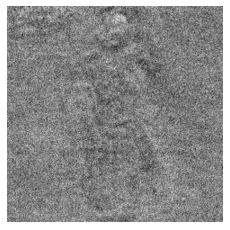3个回答cnn网络提取的图片的正数据格式是什么样的

CNN模型为AlexNet，平台是MATLAB,由于实验室数据不可外泄，在此用ABC代替 识别原理是对ABC信号进行时频分析，生成时频图像，最为CNN的输入样本 ## 由于信号A较长，将其分为3段，用其中一段（称为A1）和信号B、C组合成一个3个种类的样本集来进行训练测试，识别率良好在97%左右，当保存上述训练好的CNN网络，对信号A2和A3信号进行测试时识别正确率为0，说明A1A2A3为三个种类，CNN无法将其识别成一类，这个可以理解，可能有3段信号的特征不同，但是奇怪的来了！！！！！！！，当把A1A2A3组合成一个3个种类的样本集进行网络训练的时候，识别率只达到35%，那么对于3个种类的样本来讲，这就表示cnn不能把A1A2A3分开，说明他们是一个种类，这样的话前后矛盾了， 针对此问题求大神解答，本人研二马上要毕业，导师临时要我添加此内容，遇到问题十万火急，本人可能才疏学浅，目前确实束手无策，如有能解决的朋友也可私聊，

cnn中卷积核和特征数量的问题。

CNN卷积神经网络中过滤器每个Kernel的层数永远等于上层特征图个数吗？

CNN文本特征提取后，如何将最终提取的特征向量输出？

CNN图形识别、识别率差，该如何训练模型？

tensoflow的训练图片和预测图片尺寸必须一致吗？

# tensoflow的训练图片和预测图片尺寸必须一致吗？ ## 要预测一张尺寸比较大的图片上的所有物体应该怎么处理

Pytorch下如何实现一个cnn和非图像数据混合输入的网络

tensorflow CNN训练图片分类的时候，模型训练不出来，准确率0.1（分了十类），模型失效，是什么原因？

``` def compute_accuracy(v_xs, v_ys): global prediction y_pre = sess.run(prediction, feed_dict={xs: v_xs, keep_prob: 1}) correct_prediction = tf.equal(tf.argmax(y_pre,1), tf.argmax(v_ys,1)) accuracy = tf.reduce_mean(tf.cast(correct_prediction, tf.float32)) result = sess.run(accuracy, feed_dict={xs: v_xs, ys: v_ys, keep_prob: 1}) return result def weight_variable(shape): initial = tf.truncated_normal(shape, stddev=0.1) return tf.Variable(initial) def bias_variable(shape): initial = tf.constant(0.1, shape=shape) return tf.Variable(initial) def conv2d(x, W): # stride [1, x_movement, y_movement, 1] # Must have strides = strides = 1 return tf.nn.conv2d(x, W, strides=[1, 1, 1, 1], padding='SAME') def max_pool_2x2(x): # stride [1, x_movement, y_movement, 1] return tf.nn.max_pool(x, ksize=[1,4,4,1], strides=[1,4,4,1], padding='SAME') # define placeholder for inputs to network xs = tf.placeholder(tf.float32, [None, 65536])/255. # 256x256 ys = tf.placeholder(tf.float32, [None, 10]) keep_prob = tf.placeholder(tf.float32) x_image = tf.reshape(xs, [-1, 256, 256, 1]) # print(x_image.shape) # [n_samples, 28,28,1] ## conv1 layer ## W_conv1 = weight_variable([3,3, 1,64]) # patch 5x5, in size 1, out size 32 b_conv1 = bias_variable() h_conv1 = tf.nn.elu(conv2d(x_image, W_conv1) + b_conv1) # output size 28x28x32 h_pool1 = tf.nn.max_pool(h_conv1, ksize=[1,2,2,1], strides=[1,2,2,1], padding='SAME') # output size 14x14x32 ## conv2 layer ## W_conv2 = weight_variable([3,3, 64, 128]) # patch 5x5, in size 32, out size 64 b_conv2 = bias_variable() h_conv2 = tf.nn.elu(conv2d(h_pool1, W_conv2) + b_conv2) # output size 14x14x64 h_pool2 = max_pool_2x2(h_conv2) # output size 7x7x64 ## conv3 layer ## W_conv3 = weight_variable([3,3, 128, 256]) # patch 5x5, in size 32, out size 64 b_conv3 = bias_variable() h_conv3 = tf.nn.elu(conv2d(h_pool2, W_conv3) + b_conv3) # output size 14x14x64 h_pool3 = max_pool_2x2(h_conv3) ## conv4 layer ## W_conv4 = weight_variable([3,3, 256, 512]) # patch 5x5, in size 32, out size 64 b_conv4 = bias_variable() h_conv4 = tf.nn.elu(conv2d(h_pool3, W_conv4) + b_conv4) # output size 14x14x64 h_pool4 = max_pool_2x2(h_conv4) # ## conv5 layer ## # W_conv5 = weight_variable([3,3, 512, 512]) # patch 5x5, in size 32, out size 64 # b_conv5 = bias_variable() # h_conv5 = tf.nn.relu(conv2d(h_pool3, W_conv4) + b_conv4) # output size 14x14x64 # h_pool5 = max_pool_2x2(h_conv4) ## fc1 layer ## W_fc1 = weight_variable([2*2*512, 128]) b_fc1 = bias_variable() # [n_samples, 7, 7, 64] ->> [n_samples, 7*7*64] h_pool4_flat = tf.reshape(h_pool4, [-1, 2*2*512]) h_fc1 = tf.nn.elu(tf.matmul(h_pool4_flat, W_fc1) + b_fc1) h_fc1_drop = tf.nn.dropout(h_fc1, keep_prob) ## fc2 layer ## W_fc2 = weight_variable([128, 10]) b_fc2 = bias_variable() prediction = tf.nn.softmax(tf.matmul(h_fc1_drop, W_fc2) + b_fc2) # 定义优化器和训练op loss = tf.reduce_mean(tf.nn.softmax_cross_entropy_with_logits(labels=ys, logits=prediction)) train_step = tf.train.RMSPropOptimizer((1e-3)).minimize(loss) correct_prediction = tf.equal(tf.argmax(prediction, 1), tf.argmax(ys, 1)) accuracy = tf.reduce_mean(tf.cast(correct_prediction, tf.float32)) # 用于保存和载入模型 saver = tf.train.Saver() def int2onehot(train_batch_ys): num_labels = train_batch_ys.shape num_classes=10 index_offset = np.arange(num_labels) * num_classes labels_one_hot = np.zeros((num_labels, num_classes),dtype=np.float32) labels_one_hot.flat[index_offset + train_batch_ys.ravel()] = 1 return labels_one_hot train_label_lists, train_data_lists, train_fname_lists = read_tfrecords(train_tfrecord_file) iterations = 100 with tf.Session() as sess: sess.run(tf.global_variables_initializer()) # 执行训练迭代 for it in range(iterations): # 这里的关键是要把输入数组转为np.array for i in range(200): train_label_list = train_label_lists[i] train_data_list= train_data_lists[i] train_name_list = train_fname_lists[i] #print("shape of train_data_list: {}\tshape of train_label_list: {}".format(train_data_list.shape, train_label_list.shape)) #print('该批文件名：',train_name_list) print('该批标签：',train_label_list) # 计算有多少类图片 #num_classes = len(set(train_label_list)) #print("num_classes:",num_classes) train_batch_xs = train_data_list train_batch_xs = np.reshape(train_batch_xs, (-1, 65536)) train_batch_ys = train_label_list train_batch_ys = int2onehot(train_batch_ys) #print('第'+str(i)+'批-----------') print("连接层1之后----------------------------------------") for i in range(80): print("元素"+str(i)+"：",sess.run(tf.reduce_mean(sess.run(h_fc1_drop,feed_dict={xs: train_batch_xs, ys: train_batch_ys, keep_prob: 0.5})[i].shape))) print("元素"+str(i)+"：",sess.run(h_fc1_drop,feed_dict={xs: train_batch_xs, ys: train_batch_ys, keep_prob: 0.5})[i]) print("连接层2之后----------------------------------------") for i in range(80): print("元素"+str(i)+"：",sess.run(tf.reduce_mean(sess.run(prediction,feed_dict={xs: train_batch_xs, ys: train_batch_ys, keep_prob: 0.5})[i].shape))) print("元素"+str(i)+"：",sess.run(prediction,feed_dict={xs: train_batch_xs, ys: train_batch_ys, keep_prob: 0.5})[i]) #loss.run(feed_dict={xs: train_batch_xs, ys: train_batch_ys, keep_prob: 0.5}) train_step.run(feed_dict={xs: train_batch_xs, ys: train_batch_ys, keep_prob: 0.5}) time.sleep(7) # 每完成五次迭代，判断准确度是否已达到100%，达到则退出迭代循环 iterate_accuracy = 0 if it%5 == 0: iterate_accuracy = accuracy.eval(feed_dict={xs: train_batch_xs, ys: train_batch_ys, keep_prob: 0.5}) print ('iteration %d: accuracy %s' % (it, iterate_accuracy)) if iterate_accuracy >= 1: break; print ('完成训练!') ```

tensorflow CNN训练mnist数据集后识别自己写的数字效果不好

1. 因为我只需要CNN的这个特征向量，但是我找不到从哪里打断点获取，请问哪位大神知道如何在MATLAB中获取这个CNN的特征向量呢？多谢~

keras中使用预训练的VGG19网络能否处理单通道的灰度图？

CNN神经网络数字识别样本该如何输入？

R-CNN关于网络结构的问题

1.为什么输入SVM分类器用4096维的特征，不用pool5中的特征向量。毕竟难道不是卷积层得出图像的特征，后面全连接是用来分类吗？ 2.bb regression中，输入是pool5的向量，也就是输入的size是6x6x256？ 3.pre-training和fine-tuning是用softmax+交叉熵训练吗？

Python可以这样学（第一季：Python内功修炼）

NS网络模拟和协议仿真源代码

NS网络模拟和协议仿真源代码，包含代码说明及协议分析

【超实用课程内容】 本课程演示的是一套基于Java的SSM框架实现的图书管理系统，主要针对计算机相关专业的正在做毕设的学生与需要项目实战练习的java人群。详细介绍了图书管理系统的实现，包括：环境搭建、系统业务、技术实现、项目运行、功能演示、系统扩展等，以通俗易懂的方式，手把手的带你从零开始运行本套图书管理系统，该项目附带全部源码可作为毕设使用。 【课程如何观看？】 PC端：https://edu.csdn.net/course/detail/27513 移动端：CSDN 学院APP（注意不是CSDN APP哦） 本课程为录播课，课程2年有效观看时长，大家可以抓紧时间学习后一起讨论哦~ 【学员专享增值服务】 源码开放 课件、课程案例代码完全开放给你，你可以根据所学知识，自行修改、优化

150讲轻松搞定Python网络爬虫

【为什么学爬虫？】 &nbsp; &nbsp; &nbsp; &nbsp;1、爬虫入手容易，但是深入较难，如何写出高效率的爬虫，如何写出灵活性高可扩展的爬虫都是一项技术活。另外在爬虫过程中，经常容易遇到被反爬虫，比如字体反爬、IP识别、验证码等，如何层层攻克难点拿到想要的数据，这门课程，你都能学到！ &nbsp; &nbsp; &nbsp; &nbsp;2、如果是作为一个其他行业的开发者，比如app开发，web开发，学习爬虫能让你加强对技术的认知，能够开发出更加安全的软件和网站 【课程设计】 一个完整的爬虫程序，无论大小，总体来说可以分成三个步骤，分别是： 网络请求：模拟浏览器的行为从网上抓取数据。 数据解析：将请求下来的数据进行过滤，提取我们想要的数据。 数据存储：将提取到的数据存储到硬盘或者内存中。比如用mysql数据库或者redis等。 那么本课程也是按照这几个步骤循序渐进的进行讲解，带领学生完整的掌握每个步骤的技术。另外，因为爬虫的多样性，在爬取的过程中可能会发生被反爬、效率低下等。因此我们又增加了两个章节用来提高爬虫程序的灵活性，分别是： 爬虫进阶：包括IP代理，多线程爬虫，图形验证码识别、JS加密解密、动态网页爬虫、字体反爬识别等。 Scrapy和分布式爬虫：Scrapy框架、Scrapy-redis组件、分布式爬虫等。 通过爬虫进阶的知识点我们能应付大量的反爬网站，而Scrapy框架作为一个专业的爬虫框架，使用他可以快速提高我们编写爬虫程序的效率和速度。另外如果一台机器不能满足你的需求，我们可以用分布式爬虫让多台机器帮助你快速爬取数据。 &nbsp; 从基础爬虫到商业化应用爬虫，本套课程满足您的所有需求！ 【课程服务】 专属付费社群+每周三讨论会+1v1答疑

cuda开发cutilDLL

Tensorflow与python3.7适配版本

tensorflow与python3.7匹配的最新库，更新了python3.7以后可以自行下载，或者去国外python的扩展包下载界面自行下载。

4小时玩转微信小程序——基础入门与微信支付实战

<p> 限时福利限时福利，<span>15000+程序员的选择！</span> </p> <p> 购课后添加学习助手（微信号：csdn590），按提示消息领取编程大礼包！并获取讲师答疑服务！ </p> <p> <br> </p> <p> 套餐中一共包含5门程序员必学的数学课程（共47讲） </p> <p> 课程1：《零基础入门微积分》 </p> <p> 课程2：《数理统计与概率论》 </p> <p> 课程3：《代码学习线性代数》 </p> <p> 课程4：《数据处理的最优化》 </p> <p> 课程5：《马尔可夫随机过程》 </p> <p> <br> </p> <p> 哪些人适合学习这门课程？ </p> <p> 1）大学生，平时只学习了数学理论，并未接触如何应用数学解决编程问题； </p> <p> 2）对算法、数据结构掌握程度薄弱的人，数学可以让你更好的理解算法、数据结构原理及应用； </p> <p> 3）看不懂大牛代码设计思想的人，因为所有的程序设计底层逻辑都是数学； </p> <p> 4）想学习新技术，如：人工智能、机器学习、深度学习等，这门课程是你的必修课程； </p> <p> 5）想修炼更好的编程内功，在遇到问题时可以灵活的应用数学思维解决问题。 </p> <p> <br> </p> <p> 在这门「专为程序员设计的数学课」系列课中，我们保证你能收获到这些:<br> <br> <span> </span> </p> <p class="ql-long-24357476"> <span class="ql-author-24357476">①价值300元编程课程大礼包</span> </p> <p class="ql-long-24357476"> <span class="ql-author-24357476">②应用数学优化代码的实操方法</span> </p> <p class="ql-long-24357476"> <span class="ql-author-24357476">③数学理论在编程实战中的应用</span> </p> <p class="ql-long-24357476"> <span class="ql-author-24357476">④程序员必学的5大数学知识</span> </p> <p class="ql-long-24357476"> <span class="ql-author-24357476">⑤人工智能领域必修数学课</span> </p> <p> <br> 备注：此课程只讲程序员所需要的数学，即使你数学基础薄弱，也能听懂，只需要初中的数学知识就足矣。<br> <br> 如何听课？ </p> <p> 1、登录CSDN学院 APP 在我的课程中进行学习； </p> <p> 2、登录CSDN学院官网。 </p> <p> <br> </p> <p> 购课后如何领取免费赠送的编程大礼包和加入答疑群？ </p> <p> 购课后，添加助教微信：<span> csdn590</span>，按提示领取编程大礼包，或观看付费视频的第一节内容扫码进群答疑交流！ </p> <p> <img src="https://img-bss.csdn.net/201912251155398753.jpg" alt=""> </p>

MFC一站式终极全套课程包

C++语言基础视频教程

C++语言基础视频培训课程：本课与主讲者在大学开出的程序设计课程直接对接，准确把握知识点，注重教学视频与实践体系的结合，帮助初学者有效学习。本教程详细介绍C++语言中的封装、数据隐藏、继承、多态的实现等入门知识；主要包括类的声明、对象定义、构造函数和析构函数、运算符重载、继承和派生、多态性实现等。 课程需要有C语言程序设计的基础（可以利用本人开出的《C语言与程序设计》系列课学习）。学习者能够通过实践的方式，学会利用C++语言解决问题，具备进一步学习利用C++开发应用程序的基础。

Java8零基础入门视频教程

HoloLens2开发入门教程

C/C++学习指南全套教程

C/C++学习的全套教程，从基本语法，基本原理，到界面开发、网络开发、Linux开发、安全算法，应用尽用。由毕业于清华大学的业内人士执课，为C/C++编程爱好者的教程。

pokemmo的资源

pokemmo必须的4个rom 分别为绿宝石 火红 心金 黑白 还有汉化补丁 资源不错哦 记得下载

Java面试史上最全的JAVA专业术语面试100问 (前1-50)

2019 AI开发者大会

2019 AI开发者大会(AI ProCon 2019)是由中国IT社区CSDN主办的AI技术与产业年度盛会。多年经验淬炼，如今蓄势待发：2019年9月6-7日，大会将有近百位中美顶尖AI专家、知名企业代表以及千余名AI开发者齐聚北京，进行技术解读和产业论证。我们不空谈口号，只谈技术，诚挚邀请AI业内人士一起共铸人工智能新篇章！

linux“开发工具三剑客”速成攻略

DirectX修复工具V4.0增强版

DirectX修复工具(DirectX Repair)是一款系统级工具软件，简便易用。本程序为绿色版，无需安装，可直接运行。 本程序的主要功能是检测当前系统的DirectX状态，如果发现异常则进行修复

20行代码教你用python给证件照换底色

20行代码教你用python给证件照换底色

2019 Python开发者日-培训